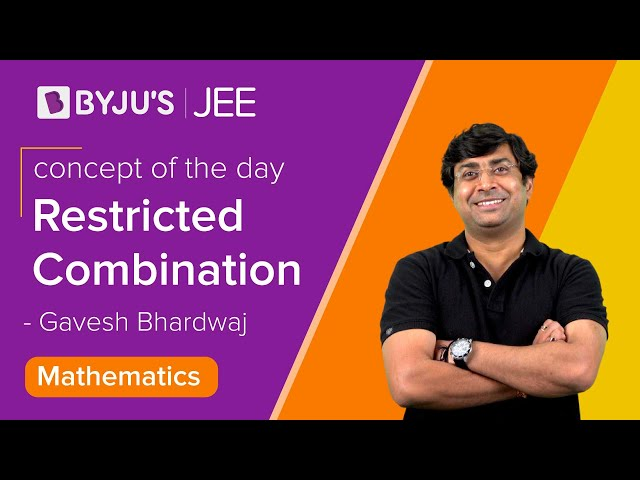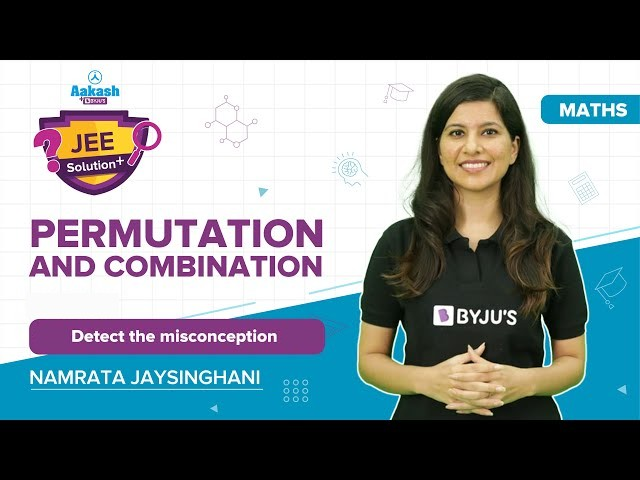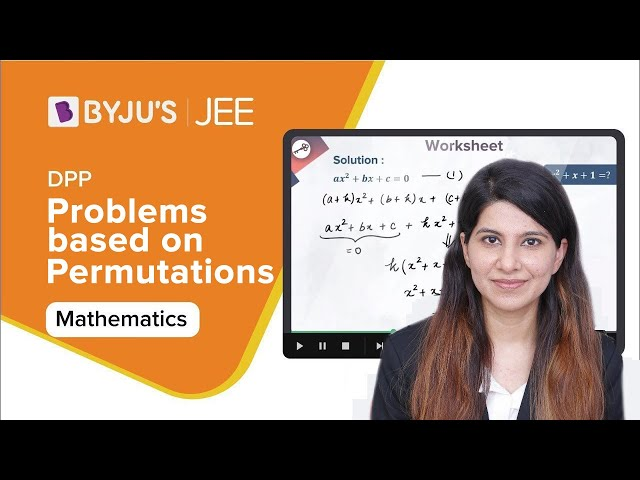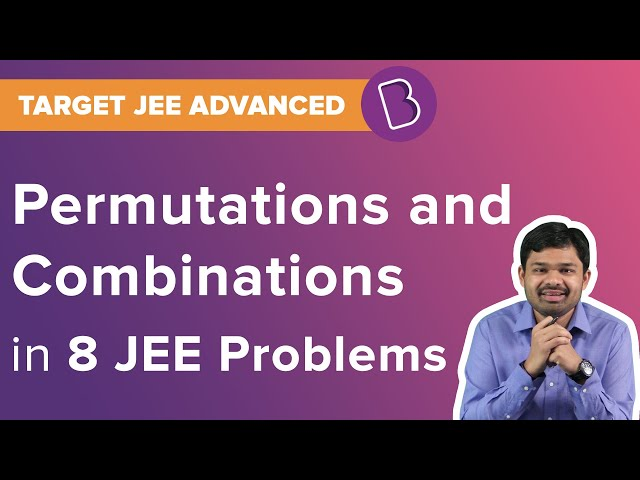# Permutations and Combinations

In real life, we are fascinated with numbers and counting the numbers. Suppose, you have written 5 letters and the letters will be put into 5 different envelopes. But one of them will be faulty i.e., the letter will not be put into the correct envelope and as a result, the letter will not reach the intended person correctly. Can we guess in how many ways the correct letter can be put into the wrong envelope? Here comes the concept of permutation and combination. Basically, we are counting numerous ways through which this can be solved.

 There are two basic principles of counting are: Fundamental principle of counting: Let the event X occurs in n different ways and another event Y occurs in m different ways. The total number of occurrences of two events is m x n. Addition principle: Let the event X occurs in m different ways and event Y occurs in n different ways and both the event cannot occur together, so, the occurrence of events X or Y is m + n.

## What is Permutation in Maths?

A permutation means, a number of objects arranged in a definite order by taking some numbers or all numbers at one time.

When all objects are distinct, we arrange the numbers by:

 The permutation value by taking r objects out of n objects at a time will be represented by n(n -1)(n – 2)(n – 3)………….(n – r + 1) which is written as nPr which can also be written as $\frac{n!}{(n-r)!}$ Thus, $^nP_0 = \frac{n!}{(n-0)!}=\frac{n!}{n!} = 1$   $^nP_n = \frac{n!}{(n-n)!}=\frac{0!}{0!} = 1$ So, permutation is same as counting some numbers by keeping other numbers constant.

When all objects are not distinct:

 Suppose, we have the word MADAM. If we do the permutation of the word, we will get $\frac{5!}{2!} = \frac{120}{4} = 30$ because we can see that the first letter ‘M’ and the first letter ‘A’ are the same as that of the second letter ‘M’ and ‘A’.

When out of n objects, r objects are same the rest are different:

 The permutation in such case will be given by $\frac{n!}{p!}$

When out of n objects, k1 objects are of the same kind, k2 objects are of another kind, k3 objects are of another kind…ka is of a-th kinds, then

 The permutation will be given by: $\frac{n!}{k_1! \times k_2! \times k_3! \times …. \times k_a! }$

#### Circular Permutations#### Permutation with Repetition## What is Combination in Maths?

Selection of objects from a group of objects where the order of selection does not matter.

Selection of r objects from n objects is given by:

$^nC_r = \frac{n!}{r!(n-r)!}$

#### Formulas for Combinations#### Restricted Combination## Solved Examples on Permutation and Combination

Example 1:

How many numbers greater than 2000 but less than 5000 can be formed by digits 0,1,2,3,4,5,6 and 7 with a) repetition and b) without repetition will be?

Solution:

In the first place with repetition, we can arrange the number as 2,3 and 4 only.

The other three places can be filled in 8 x 8 x 8 = 512 ways. SO, the total numbers with repetition will be: 3 x 8 x 8 x 8 = 3 x 512 = 1536 ways.

Without repetition

The first place can be filled by again 2,3 and 4.

The remaining three places can be filled by 7 x 6 x 5 = 210 way.

So, the total number of ways will be without repetition will be: 3 x 7 x 6 x 5 = 630 ways.

Example 2:

If all the words of Rose are arranged and written in alphabetical order, what will be the position of Rose in that?

Solution:

Word starting with E: 4! = 24 ways

Words starting with O: 4! = 24 ways

Word starting with R and E: 2! = 2 ways

Word starting with R and O: First word is ROES and the Second word will be ROSE.

So, the number of ways will be: 24 + 24 + 2 + 2 = 52 ways.

Example 3:

(a) In how many ways can you allot 5 beds to 3 people?

(b) In how many ways can the letters of the word ‘MOBILE’ be arranged?

Solution:

(a) First person can choose any one bed among the 5 beds available.

Similarly, 2nd person will be left with 4 choices and 3rd will be having 3 choices.

Number of ways of allotting = 5 x 4 x 3 = 60 ways

(b) Letters of the word ‘MOBILE’ can be arranged in 5! Ways i.e.120 ways.

Example 4: There are 10 points out of which 3 are collinear. Find: How many lines can be formed by using these points?

Solution:

Since to form one line two points are required.

Let’s suppose all the points are non-collinear, number of lines formed using these points will be = 10C2.

And number of lines formed using collinear points will be = 3C2.

Therefore, number of lines formed will be = 10C23C2 + 1 (where 1 is for the one line that is being formed using those 3 points).

Example 5: In how many ways 5 boys can be arranged in a queue such that:

(a) Two particular boys of them are always together

(b) Two particular boys of them are never together

Solution:

(a) If two boys are always together, two boys will be treated as one entity. Hence it can be arranged in 4! ways.

Again, two as one entity can also arrange among themselves in 2 ways.

Therefore, required arrangement = 4! x 2 = 24 x 2 = 48 ways.

(b) Among the 5 boys, total number of permutations will be = 5! = 120.

The arrangement in which two boys are always together is 48 ways.

The remaining in no two boys are never together will be = 120 – 48 = 72 ways.

Also Know:

De-arrangement: It can be defined as the permutation arrangement of elements of a certain in such a way that no element of that set appears in original positions. It is given by the formula given below.

$D_n = n! (1 – \frac{1}{1!} + \frac{1}{2!} – \frac{1}{3!} + \frac{1}{4!} – \frac{1}{5!} + \frac{1}{6!}…………… \frac{1}{n!})$

Example 6: How many combinations can be made up of 4 birds, 5 amphibians and 3 reptiles so that each combination has birds, amphibians and reptiles?

Solution

Since it asks the case of number of combinations i.e. at least 1 of each category among birds, amphibians and reptiles.

So combination of birds is given by = 24-1.

Similarly the combination of amphibians is given by = 25-1.

The combination of reptiles is given by = 23 – 1.

Therefore, the total combination at least one of each category will be = (24 – 1) x (25 – 1) x (23 – 1) = 3255.

Example 7: How many different (i) combination (ii) permutation can be made by using four letters of the word “COMBINATION”

Solution:

(i)

Since in the word “COMBINATION” there are 2O, 2I and 2N. Hence, there are 8 distinct words in letter. For combination selection has to be made. 4 letter words can be selected in 3 different ways which are:-

Case 1: All are 4 are different letters: There 8 distinct letters hence selection can be made in = 8C4 = 70

Case 2: 2 are same letters and 2 are different letters. There are 3 pair of two same letters from which 1 pair has to be selected and rest two are selected from 7 remaining. Thus, selection can be made in = 3C1 x 7C3 = 63.

Case 3: 2 are same and other 2 are also same letters: From the three pairs selection of two pairs has to be made i.e 3C2 = 3.

Therefore, Total combinations: = 70 + 63 + 3 = 136.

(ii)

Now permutations of these selected 4 letter words will be again 3 cases:

Case 1: All are 4 different letters: Selection as well as arrangement of these will be = 8C4 x 4! = 1680

Case 2: 2 are same letters and 2 are different letters = 3C1 x 7C3 x $\frac{4!}{2!}$ = = 756

Case 3: 2 are same and other 2 are also same letters = 3C2 x $\frac{4!}{2! \times 2!}$ = 18.

Therefore, Total permutations: = 1680 + 756 + 18 = 2454.

Question 8: A set has (2n + 1) elements. The number of subsets of the set that contains at most n elements is given by

Solution:
The number of subsets of the set which contain at most n elements is
$^{2n+1}{{C}_{0}}{{+}^{2n+1}}{{C}_{1}}+…..+{{\,}^{2n+1}}{{C}_{n}}=S\\ 2S=2{{(}^{2n+1}}{{C}_{0}}+{{\,}^{2n+1}}{{C}_{1}}+…..+{{\,}^{2n+1}}{{C}_{n}})\\ = {{(}^{2n+1}}{{C}_{0}}+{{\,}^{2n+1}}{{C}_{2n+1}})+{{(}^{2n+1}}{{C}_{1}}+{{\,}^{2n+1}}{{C}_{2n}})+……..+{{(}^{2n+1}}{{C}_{n}}+{{\,}^{2n+1}}{{C}_{n+1}}) \left\{\,{{\,}^{n}}{{C}_{r}}={{\,}^{n}}{{C}_{n-r}} \right\}\\ =^{2n+1}{{C}_{0}}+{{\,}^{2n+1}}{{C}_{1}}+…….+{{\,}^{2n+1}}{{C}_{2n+1}}={{2}^{2n+1}}\\ \Rightarrow S={{2}^{2n}}$

Question 9: If P (n, r) = 1680 and C (n, r) = 70, then 69n + r! =

Solution:
$P(n,r)=1680 \\ \frac{n!}{(n-r)!}=1680..(i) C(n,r)=70\\ \frac{n!}{r!\,(\,n-r)!}=70 ..(ii) \\ \frac{1680}{r!}=70 \\ \text \ From \ (i) \ and \ (ii), r!=\frac{1680}{70}=24\\ r=4\\ P(n,\,4)=1680\\ n(n-1)(n-2)(n-3)=1680\\ n=8\\ 8\times 7\times 6\times 5=1680\\ \text \ Now \ 69n+r\,!=69\times 8+4!=552+24= 576.$

Question 10: $^{47}{{C}_{4}}+\underset{r=1}{\overset{5}{\mathop{\sum }}}\,{}^{52-r}{{C}_{3}}=$

Solution:
$^{47}{{C}_{4}}+\sum\limits_{r=1}^{5}{^{52-r}{{C}_{3}}}\\ {{=}^{51}}{{C}_{3}}{{+}^{50}}{{C}_{3}}{{+}^{49}}{{C}_{3}}{{+}^{48}}{{C}_{3}}{{+}^{47}}{{C}_{3}}{{+}^{47}}{{C}_{4}}\\ {{=}^{51}}{{C}_{3}}{{+}^{50}}{{C}_{3}}{{+}^{49}}{{C}_{3}}{{+}^{48}}{{C}_{3}}{{+}^{48}}{{C}_{4}}={{}^{51}}{{C}_{4}}$ [Using property, $^nC_r + ^nC_{r-1} = ^{n+1}C_r$]

Question 11: $\sum\limits_{r=0}^{m}{^{n+r}{{C}_{n}}=}$

Solution:
Since $^{n}{{C}_{r}}{{=}^{n}}{{C}_{n-r}} \text \ and \ ^{n}{{C}_{r-1}}{{+}^{n}}{{C}_{r}}{{=}^{n+1}}{{C}_{r}}$, we have
$\sum\limits_{r=0}^{m}{^{n+r}{{C}_{n}}}=\sum\limits_{r=0}^{m}{^{n+r}{{C}_{r}}}{{=}^{n}}{{C}_{0}}{{+}^{n+1}}{{C}_{1}}{{+}^{n+2}}{{C}_{2}}+……{{+}^{n+m}}{{C}_{m}}\\ =[1+(n+1)]{{+}^{n+2}}{{C}_{2}}{{+}^{n+3}}{{C}_{3}}+……..{{+}^{n+m}}{{C}_{m}}\\ {{=}^{n+m+1}}{{C}_{n+1}}, [{{\ }^{n}}{{C}_{r}}{{=}^{n}}{{C}_{n-r}}]$

#### Permutations and Combination Problems and Solutions#### Problems based on Permutations and Combinations#### JEE Permutations and Combinations Solved Questions